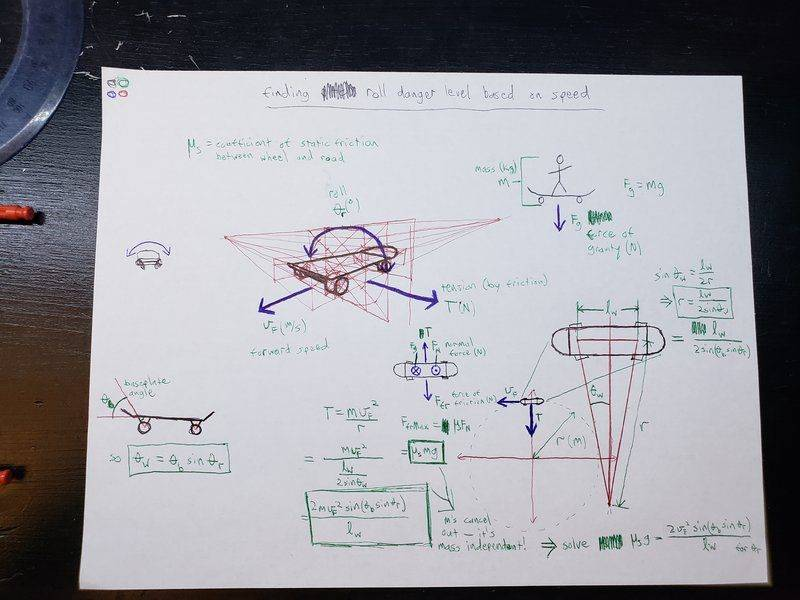# Finding the max angle of a longboard deck before the wheels slip

Hi, I'm making an electric longboard and trying to write an app for my phone to function as the remote. I've got a bunch of fancy Star-Trek-esque indicators on it, one of which is the pitch and roll of the deck. All the indicators have "danger zones" and turn red when they hit them, and for this the danger zone will be dynamic as your speed changes so I'm trying to find the maximum angle (roll) in degrees that the board can be tilted to turn before the wheels slip on the road based on speed. I thought I had it down, but must have done something wrong because the results don't quite make sense so here's my process (sorry for the picture being so scatter-brained haha)So, the board turns in a circle whose radius r changes as some function of the roll, θr. More directly, the radius depends on the angle of the wheels with the midline of the deck, θw (see lower right section of picture for clarification). One can calculate this correlation based on the length between the trucks, lw by using SOH (sin = opposite/adjacent) to get
sinθw = lw / 2r ⇒ r = lw/2sinθw
To get θw as a function of θr, I figured out it's a sinusoidal relationship to the baseplate angle θb, specifically
θw = θbsinθr
Therefore, the radius of the curve is
r = lw / 2sin(θbsinθr).

Now, to get the centrifugal force, the formula is mv2 / r, so we can simply plug in what we got for r to get
Fcen = mv2 / (lw / 2sin(θbsinθr))
which can simplify to
Fcen = 2mv2sin(θbsinθr) / lw

The force that has to counteract that is the force of friction between the wheels and the road (with axis of movement parallel to wheel's axis of rotation), the maximum of which is Ffric = μmg. Therefore to figure out the maximum θr (the end goal of all this), we need to
solve Ffric = Fcen for θr

Oh boy, here we go.
μmg = 2mv2sin(θbsinθr) / lw
First of all, the m's cancel out, meaning it's apparently mass independent (makes sense; a heavier person will produce more centrifugal force but also push harder on the ground increasing friction), so now let's just algebra the crap outta this:
μglw = 2v2sin(θbsinθr)
μglw / 2v2 = sin(θbsinθr)
sin-1glw / 2v2) = θbsinθr
sin-1glw / 2v2) / θb = sinθr
sin-1(sin-1glw / 2v2) / θb) = θr
Therefore,
θrMax = sin-1(sin-1glw / 2v2) / θb)

This should be the end of the problem, but when I applied it to the program and plugged in appropriate values for all the variables, it wasn't behaving properly (for example, when the coefficient of friction is set to 1 the maximum roll should be 90 regardless of speed but it still changes as the speed changes). Any ideas on what I might've done wrong? Do I need to bring calculus into this somewhere as an optimization problem? I'm at a loss...

#### Attachments

•berkeman

Bystander
Homework Helper
Gold Member
Are the wheels "crowned?" Any cylinder/roller/wheel with a non-zero width is going to be slipping all the time in a turn.

Are the wheels "crowned?" Any cylinder/roller/wheel with a non-zero width is going to be slipping all the time in a turn.
I don't think they're crowned if I understand that term correctly... I haven't actually purchased or built anything yet, I'm just getting the software down first and will put in values later. I realize that the wheels are slipping during a turn due to the geometry of how it works and therefore the curve itself can't be perfectly measured with this method, but I'm only trying to calculate the point at which it will cross from that required direct slippage into centrifugal force induced skidding. Maybe I am going about this entirely the wrong way though... Is there a better approach...?

A.T.
when the coefficient of friction is set to 1 the maximum roll should be 90 regardless of speed
Why?

Why?
Might be missing something here, but if the coefficient of friction is 1, doesn't that mean the surfaces have infinite friction between them (cannot move no matter how much force is applied)? And if that's the case, the maximum amount you could tilt the board to steer should be the maximum amount possible in its range of motion, which theoretically is 90°. In practice it would be less than that due to collisions, but mathematically speaking the maximum roll relative to exactly flat is exactly sideways.

A.T.
Might be missing something here, but if the coefficient of friction is 1, doesn't that mean the surfaces have infinite friction between them (cannot move no matter how much force is applied)?
No.

Ok... Care to elaborate? What DOES a coefficient of 1 mean?

A.T.
•